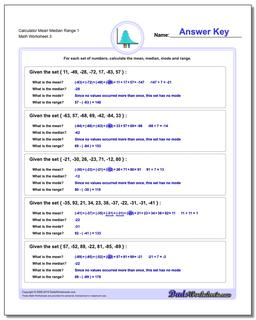# Math Worksheets: Mean, Median, Range: Mean, Median, Range: Calculator Mean Median Range 1 (Third Worksheet)## Calculator Mean Median Range 1 (Third Worksheet)

PropertyValue
DescriptionCalculator Mean Median Range 1: Harder Mixed Sign problems suitable for calculator practice. (Third Worksheet)
Resource TypeWorksheet